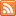Content Tagged “Probability Distribution”Did I Get This – Binomial Distribution

Published: Feb 3rd, 2013

A Gallup Poll in May 2004 estimated that roughly 70% of U.S. adults are in favor of the death penalty for a person convicted of murder. A random sample of […]

Learn by Doing – Binomial Application

Published: Feb 3rd, 2013

The purpose of this activity is to gain experience making probabilistic decisions using binomial random variables. Background In vitro fertilization is becoming more and more common these days. Suppose each […]

Learn by Doing – Binomial Probabilities

Published: Feb 3rd, 2013

The purpose of this activity is to give guided practice at solving problems involving binomial random variables and to teach how the same probabilities can be found using an online […]

Did I Get This – Counting Outcomes

Published: Feb 3rd, 2013

This document is linked from Binomial Random Variables.Binomial Random Variables

Published: Feb 3rd, 2013

Binomial Experiment Binomial Probability Distribution – Using Probability Rules Counting Outcomes Mean and Standard Deviation of the Binomial Random Variable CO-6: Apply basic concepts of probability, random variation, and commonly used […]Learn by Doing – Unusual or Not?

Published: Feb 3rd, 2013

Recall the probability distribution for changing majors. We have made the following calculations for the mean and standard deviation. For some extra practice, feel free to verify our calculations. This […]Did I Get This – Standard Deviation of a Discrete Random Variable #2

Published: Feb 3rd, 2013

This document is linked from Discrete Random Variables.

Learn by Doing – Comparing Probability Distributions #3

Published: Feb 3rd, 2013

Xavier’s Production Line: Yves’ Production Line: Hint This document is linked from Discrete Random Variables.Learn by Doing – Comparing Probability Distributions #2

Published: Feb 2nd, 2013

Keeping in mind that the mean describes where a histogram is centered, and the standard deviation describes spread by reporting the typical distance of values from their mean, match the random […]Did I Get This – Standard Deviation of a Discrete Random Variable

Published: Feb 2nd, 2013

Here again is the probability distribution of Y—the number of defective parts in an hour in Yves’ production line: Consider the following expressions: This document is linked from Discrete Random Variables.